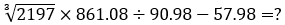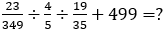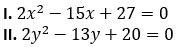Dear Aspirants,

### Quantitative Aptitude Quiz For IBPS PO/Clerk Prelims

Numerical Ability or Quantitative Aptitude Section has given heebie-jeebies to the aspirants when they appear for a banking examination. As the level of every other section is only getting complex and convoluted, there is no doubt that this section, too, makes your blood run cold. The questions asked in this section are calculative and very time-consuming. But once dealt with proper strategy, speed, and accuracy, this section can get you the maximum marks in the examination. Following is the Quantitative Aptitude quiz to help you practice with the best of latest pattern questions.
WATCH VIDEO SOLUTIONS HERE

Directions (1-5): What will come at the place of question mark in the following questions? (You are not expected to find exact value)

Q1. 449.98 ÷ 5.99 + (2.99)⁴ - 74.97 = ?
71
81
86
75
91

Q2.55
52
65
61
59

Q3. 69.98% of 259.98 – 29.98% of 529.98 = ?
19
23
20
27
18

Q4.23
19
28
24
35

Q5.489
476
550
525
500

Directions (6-7): Read the sentence below and solve the questions based on it. Two fair dices are thrown. Find the probability of getting.

Q6. A sum of two numbers divisible by 4.
1/2
3/4
1/3
2/3
1/4

Q7. A sum of two numbers to be a prime number less than 10.
11/13
1/13
1/4
13/36
23/36
Solution:Favourable cases = (1 & 1), (1 & 2), (1 & 4), (1 & 6), (2 & 1), (2 & 3), (2 & 5), (3 & 2), (3 & 4), (4 & 1), (4 & 3), (5 & 2), (6 & 1) = 13

Q8. A man bought 80 eggs at the rate of 24 rupees per dozen 10 eggs got broken and the remaining eggs he sold at 3.20 rupees per egg. If he had spent 16 rupees on other expenses, find his profit or loss percentage.

Directions (9-10): Read the passage below and solve the questions based on it.

Prabhat has certain number of Pens. Harshit bought 25% of the Pens and Avinash bought one-third of remaining Pens, Prakash bought 50% of remaining and Akash bought 4 Pens.

Q9. How many Pens did Avinash purchase?
3
4
6
8
10

Q10. How many number Pens were with Prabhat initially?
3
4
6
8
16

Directions (11-15): In the following questions there are two equations in x and y are given. Solve the following equations and give answer

Q11.if x > y
if x < y
if x ≥ y
if No relation can be established between x and y or x = y
if x ≤ y

Q12.if x > y
if x < y
if x ≥ y
if No relation can be established between x and y or x = y
if x ≤ y

Q13.if x > y
if x < y
if x ≥ y
if No relation can be established between x and y or x = y
if x ≤ y

Q14.if x > y
if x < y
if x ≥ y
if No relation can be established between x and y or x = y
if x ≤ y

Q15.if x > y
if x < y
if x ≥ y
if No relation can be established between x and y or x = y
if x ≤ y

You May also like to Read: